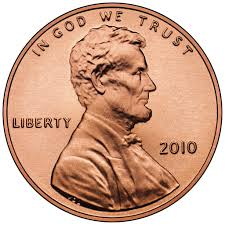Probability Level 4Another coin toss problem!

A fair coin is tossed $10$ times. What is the probability that two heads do not occur consecutively? Express the probability as a decimal.

This problem is not original. This is a hard problem, roughly at the difficulty level of a problem in the BMO. This problem is part of this set.

×